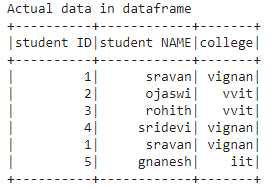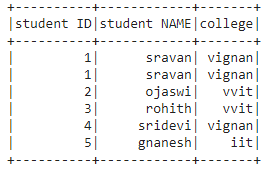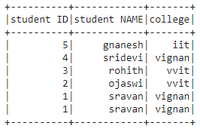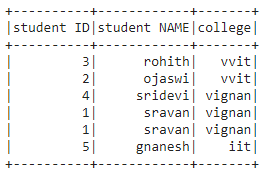Open in App
Not now

# How to Order Pyspark dataframe by list of columns ?

• Last Updated : 07 Nov, 2022

In this article, we are going to apply OrderBy with multiple columns over pyspark dataframe in Python. Ordering the rows means arranging the rows in ascending or descending order.

Method 1: Using OrderBy()

OrderBy() function is used to sort an object by its index value.

Syntax: dataframe.orderBy([‘column1′,’column2′,’column n’], ascending=True).show()

where,

• dataframe is the dataframe name created from the nested lists using pyspark
• where columns are the list of columns
• ascending=True specifies order the dataframe in increasing order, ascending=Falsespecifies order the dataframe in decreasing order
• show() method id used to display the columns.

Let’s create a sample dataframe

## Python3

 `# importing module``import` `pyspark` `# importing sparksession from pyspark.sql module``from` `pyspark.sql ``import` `SparkSession` `# creating sparksession and giving an app name``spark ``=` `SparkSession.builder.appName(``'sparkdf'``).getOrCreate()` `# list  of students  data``data ``=` `[[``"1"``, ``"sravan"``, ``"vignan"``], [``"2"``, ``"ojaswi"``, ``"vvit"``],``        ``[``"3"``, ``"rohith"``, ``"vvit"``], [``"4"``, ``"sridevi"``, ``"vignan"``],``        ``[``"1"``, ``"sravan"``, ``"vignan"``], [``"5"``, ``"gnanesh"``, ``"iit"``]]` `# specify column names``columns ``=` `[``'student ID'``, ``'student NAME'``, ``'college'``]` `# creating a dataframe from the lists of data``dataframe ``=` `spark.createDataFrame(data, columns)` `print``(``"Actual data in dataframe"``)``# show dataframe``dataframe.show()`

Output:## Python3

 `# show dataframe by sorting the dataframe``# based on two columns in ascending``# order using orderby() function``dataframe.orderBy([``'student ID'``, ``'student NAME'``],``                  ``ascending``=``True``).show()`

Output:## Python3

 `# show dataframe by sorting the dataframe``# based on two columns in descending``# order using orderby() function``dataframe.orderBy([``'student ID'``, ``'student NAME'``],``                  ``ascending``=``False``).show()`

Output:Method 2: Using sort()

It takes the Boolean value as an argument to sort in ascending or descending order.

Syntax: dataframe.sort([‘column1′,’column2′,’column n’],ascending=True).show()

where,

1. dataframe is the dataframe name created from the nested lists using pyspark
2. where columns are the llst of columns
3. ascending=True specifies order the dataframe in increasing order,ascending=Falsespecifies order the dataframe in decreasing order
4. show() method id used to display the columns.

## Python3

 `# show dataframe by sorting the dataframe``# based on two columns in descending order``dataframe.sort([``'college'``, ``'student NAME'``], ascending``=``False``).show()`

Output:My Personal Notes arrow_drop_up